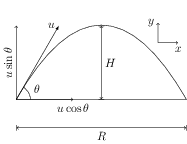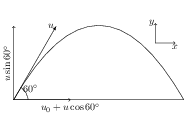# Projectiles

## Concepts and FormulasLet a particle be projected from a point O with an initial velocity $u$ at an angle $\theta$ with the horizontal. The motion lies in a vertical plane containing the point O and the initial velocity vector. Let $x$ be the horizontal and $y$ be the vertical directions in this plane. If we take O as the origin $(x=0,y=0)$ and the instant of projection as time $t=0$ then initial position, velocity, and acceleration of the particle are given by, \begin{align} x&=0, && u_x&=u\cos\theta, && a_x&=0; \\ y&=0, && u_y&=u\sin\theta, && a_y&=-g; \end{align} where $g$ is the acceleration due to gravity. Use equation of motion, $s=ut+\frac{1}{2}at^2$, to get the position at the time $t$ as, \begin{align} x=ut\cos\theta,&& y=ut\sin\theta-\tfrac{1}{2}gt^2. \end{align} Eliminate $t$ from the expressions of $x$ and $y$ to get the equation of trajectory, \begin{align} y=x\tan\theta-\frac{g}{2u^2\cos^2\theta} x^2, && \text{(equation of a parabola)}. \end{align}

The time of flight is given by \begin{align} T=\frac{2u\sin\theta}{g}, \end{align}

and the range is \begin{align} R=\frac{u^2\sin2\theta}{g}. \end{align} The maximum range for given initial velocity is $R_\text{max}=u^2/g$ and it occurs at $\theta=45^\mathrm{o}$.

The maximum height of the projectile is given by \begin{align} H=\frac{u^2 \sin^2 \theta}{2g}. \end{align} Note that $v_y=0$ at maximum height.

## Problems from IIT JEE

Problem (IIT JEE 2011): A train is moving along straight line with a constant acceleration $a$. A boy standing in the train throws a ball forward with a speed of 10 m/s at an angle ${60}^\mathrm{o}$ from horizontal. The boy has to move forward by 1.15 m inside the train to catch the ball back at the initial height. The acceleration of the train in $\mathrm{m/s^2}$ is,

Solution:Consider the motion in a frame attached to the ground. Let train's velocity at the time of projection of ball be $u_0$. The ball is projected with a velocity $u={10}\;\mathrm{m/s}$ (relative to the train) at an angle ${60}^\mathrm{o}$ from the horizontal (see figure). Thus the horizontal and vertical component of the initial velocity of the ball are, \begin{alignat}{2} &u_{x}=u_0+u\cos 60, \nonumber\\ & u_{y}=u\sin 60. \nonumber \end{alignat} The time of flight is given by $t=2u_y/g=2u\sin 60\,/g=\sqrt{3}\;\mathrm{s}$. The distance travelled by the train and the ball in time $t$ are $x_\text{train}=u_0 t+\frac{1}{2}a t^2$ and $x_\text{ball}=(u_0+u\cos 60) t$. Since the boy moves forward by 1.15 m, we have, \begin{alignat}{2} & x_\text{ball}=x_\text{train}+1.15, \nonumber\\ \label{gba:eqn:1} & (u_0+u\cos 60) t=u_0 t+\tfrac{1}{2}a t^2+1.15. \end{alignat} Substitute $u={10}\;\mathrm{m/s}$ and $t=\sqrt{3}\;\mathrm{s}$ in first equation and then solve to get $a={5}\;\mathrm{m/s^2}$. The readers are encouraged to solve this problem in a frame attached to the train.